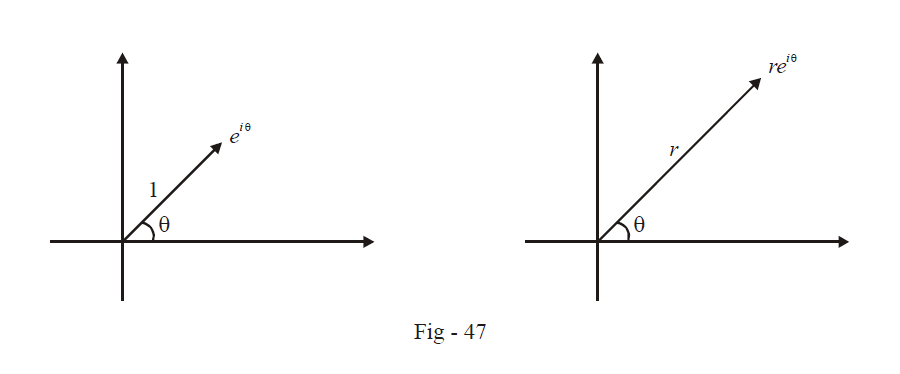# Eulers Form of Complex Numbers

Go back to  'Complex Numbers'

Learn from the best math teachers and top your exams

• Live one on one classroom and doubt clearing
• Practice worksheets in and after class for conceptual clarity
• Personalized curriculum to keep up with school

The Euler’s form of a complex number is important enough to deserve a separate section. It is an extremely convenient representation that leads to simplifications in a lot of calculations. Euler’s representation tells us that we can write $$\cos \theta + i\sin \theta \,\,{\rm{as}}\,\,{e^{i\theta }}$$

${e^{i\theta }} = \cos \theta + i\sin \theta$

where $$e$$ is the natural base introduced in the unit on Functions $$\left( {e\,\,{\rm{is}}\,\,{\rm{actually}}\mathop {\lim }\limits_{x \to 0} {{\left( {1 + x} \right)}^{1/x}}} \right)$$

This representation might seem confusing at first. What sense does it make to raise a real number to an imaginary number? However, you may rest assured that a valid justification for this relation exists.  Although we will not discuss a rigorous proof for this, you may observe the following approximate proof to see why it should be true.

We use the following expansion series for  $${e^x}$$ :

${e^x} = 1 + x + \frac{{{x^2}}}{{2!}}\; + \frac{{{x^3}}}{{3!}} + \frac{{{x^4}}}{{4!}} + ...\infty$

Now, we assume that this expansion holds true even if $$x$$ is a non-real number. In a rigorous proof, even this assumption will have to be justified, but for now, let us take its truth to be granted, and use $$x = i\,\theta$$.

\begin{align}{e^{i\,\theta }} &= 1 + i\,\theta + \frac{{{i^2}{\theta ^2}}}{{2!}} + \frac{{{i^3}{\theta ^3}}}{{3!}} + \frac{{{i^4}{\theta ^4}}}{{4!}} + ...\infty \\& = 1 + i\,\theta - \frac{{{\theta ^2}}}{{2!}} - \frac{{i\,{\theta ^3}}}{{3!}} + \frac{{{\theta ^4}}}{{4!}} + ...\infty \\&= \left( {1 - \frac{{{\theta ^2}}}{{2!}} + \frac{{{\theta ^4}}}{{4!}} - ...\infty } \right) + i\left( {\theta - \frac{{{\theta ^3}}}{{3!}} + \frac{{{\theta ^5}}}{{5!}} - ...\infty } \right)\end{align}

The two series are (Taylor) expansion series for $$\cos \theta$$ and $$\sin \theta$$ thus

${e^{i\theta }} = \cos \theta + i\sin \theta$

Let us now use this relation for a few examples:

*    $$z = 1$$ can be written as $$e{i^0}$$

*     $$z = i$$ and $$z = – i$$ can be written as $${e^{i\pi /2}}\,{\rm{and}}\,\,{e^{ - i\pi /2}}$$ respectively

*     $$z = –1$$ can be written as $${e^{i\pi }}$$

*   Any arbitrary complex number $$z$$ can be written as $$r\left( {\cos \theta + i\sin \theta } \right)$$ or $$r{e^{i\theta }}$$ where $$r$$ is the modulus and $$\theta$$ the argument of $$z.$$

*   Multiplication and division become a whole lot easier

Let $${z_1} = {r_1}{e^{i{\theta _1}}}\,\,\,{\rm{and}}\,\,{z_2} = {r_2}{e^{i{\theta _2}}}$$

$\Rightarrow {z_1}{z_2} = {r_1}{e^{i{\theta _1}}}\,.\,\,{r_i}{e^{i{\theta _2}}} = {r_1}{r_2}{e^{i\left( {{\theta _1} + {\theta _2}} \right)}}$

This result is in agreement with the fact that moduli multiply and arguments add  upon multiplication

$\Rightarrow \frac{{{z_1}{\kern 1pt} }}{{{z_2}}} = \frac{{{r_1}{e^{i{\theta _1}}}}}{{{r_2}{e^{i{\theta _2}}}}} = \frac{{{r_1}}}{{{r_2}}}{e^{\,i\left( {{\theta _1} - {\theta _2}} \right)}}$

As in multiplication the relation above confirms the corresponding property of division of complex numbers.

From now onwards, whenever working in the polar form, we will use the Euler representation

Also, some readers might have noticed by now that addition/subtraction is more convenient in Cartesian form (where we add/subtract the real and imaginary components separately) while multiplication/division is generally more convenient in Polar (Euler) form where we deal simply with multiplication/division and addition/subtraction of moduli and arguments respectively.

Before concluding, we would like to, for the sake of emphasis, repeat this important fact about Euler’s representation again $${e^{i\theta }}$$  which is $$\cos \theta + i\sin \theta ,$$  represents a unit vector making an angle $$\theta$$  with the positive x-axis $$r{e^{i\theta }}$$;  represents a vector of magnitude $$r$$ at an angle $$\theta$$ with the positive $$x$$-axis.Therefore, you should view  $$r{e^{i\theta }}$$ as the multiplication of a vector $${e^{i\theta }}$$, by a scalar $$r,$$ which has the effect of modifying the magnitude of the vector while preserving its direction

## TRY YOURSELF - VI

Q. 1 Write the Euler’s form for the following:

(a) $$- \sqrt 2$$

(b) \begin{align} - \frac{1}{2} + i\frac{{\sqrt 3 }}{2}\end{align}

(c) \begin{align}\frac{1}{{\sqrt 2 }} + \frac{1}{{\sqrt 2 }}\;i\end{align}

(d) $$\pi - \pi i$$

Q. 2 Use the Euler’s form of $$i$$ to evaluate $${i^i}$$ .

Learn from the best math teachers and top your exams

• Live one on one classroom and doubt clearing
• Practice worksheets in and after class for conceptual clarity
• Personalized curriculum to keep up with school

0